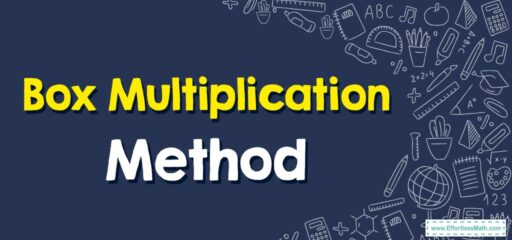# How to Use Box Multiplication Method

The Box Multiplication Method, also known as the Area Model, is a method to multiply large numbers visually. This can be particularly helpful in understanding multiplication conceptually, especially for those who are visual learners. It's also a common tool used in teaching the Common Core method of mathematics.## A Step-by-step Guide to Using Box Multiplication Method

Here’s a step-by-step guide to using the Box Multiplication Method:

Let’s say we want to multiply 23 by 45.

### Step 1: Draw a box

Start by drawing a large rectangle or box on your paper. Then divide this box into four smaller boxes by drawing one vertical line and one horizontal line.

### Step 2: Label the boxes

Write the first number (23) on the top of the box, splitting the digits above the vertical lines. So, you will write ‘2’ above the left-hand box and ‘3’ above the right-hand box.

Do the same with the second number (45) on the side of the box, writing ‘4’ next to the top box and ‘5’ next to the bottom box.

### Step 3: Multiply and fill in the boxes

Now, you’ll multiply the numbers corresponding to each box and fill in the box with the result. The top left box will be the multiplication of ‘2’ (from 23) and ‘4’ (from 45), which equals 8. The top right box will be the multiplication of ‘3’ (from 23) and ‘4’ (from 45), which equals 12.

The bottom left box will be the multiplication of ‘2’ (from 23) and ‘5’ (from 45), which equals 10. The bottom right box will be the multiplication of ‘3’ (from 23) and ‘5’ (from 45), which equals 15.

### Step 4: Add the results

Finally, add all the results from the boxes together. This will give you the answer to the multiplication problem. In this case, 8 + 12 + 10 + 15 = 45.

### Step 5: Adjust for place value

Remember that the numbers above and to the side of the box represent tens and ones. So the number in the top left box really represents 80 (8 tens), and the number in the bottom left box represents 100 (10 tens).

So, the final calculation should be 80 (from the top left box) + 120 (from the top right box) + 100 (from the bottom left box) + 15 (from the bottom right box) = 1035.

So, 23 multiplied by 45 equals 1035.

This method can be expanded to multiply numbers of any size, including decimals and fractions. It’s a great way to understand the principles of multiplication and the concept of place value.

### What people say about "How to Use Box Multiplication Method - Effortless Math: We Help Students Learn to LOVE Mathematics"?

No one replied yet.

X
30% OFF

Limited time only!

Save Over 30%

SAVE $5 It was$16.99 now it is \$11.99